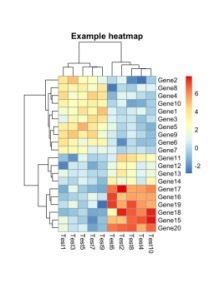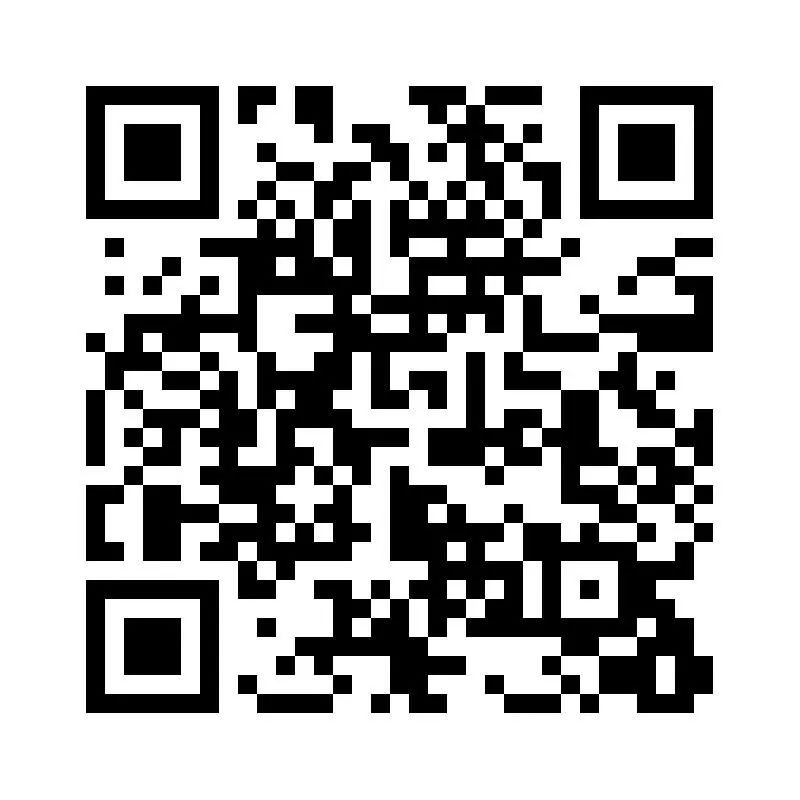## 【R语言】十分钟学会绘制聚类热图

pheatmap是R语言中一个使用非常广泛的用于绘制聚类热图的绘图包。使用这个绘图包可以帮助我们快速的生成包含聚类结果的热图。

pheatmap的安装非常简单，只需要在R软件中执行一行安装代码即可

```install.packages('pheatmap') ```

```# 加载软件包 library('pheatmap') # 生成绘图用的数据 test = matrix(rnorm(200), 20, 10) test[1:10, seq(1, 10, 2)] = test[1:10, seq(1, 10, 2)] + 3 test[11:20, seq(2, 10, 2)] = test[11:20, seq(2, 10, 2)] + 2 test[15:20, seq(2, 10, 2)] = test[15:20, seq(2, 10, 2)] + 4 colnames(test) = paste("Test", 1:10, sep = "") rownames(test) = paste("Gene", 1:20, sep = "") ```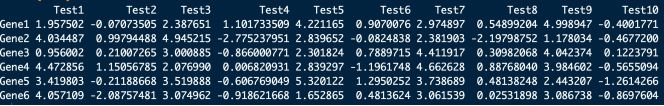`pheatmap(test)`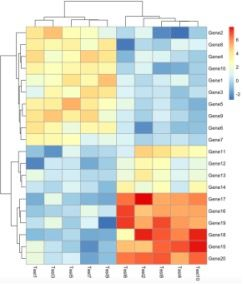```pheatmap(test, scale = "row") ```

# scale = “row”的含义是绘图时按行进行均一化。进行均一化可以降低个别特殊样品与其它样品间的差异，这会使得其它样品间的差异在图形中更加显著。一般我们在基于表达量进行聚类分析时，均是常用的参数。绘图结果如下图所示：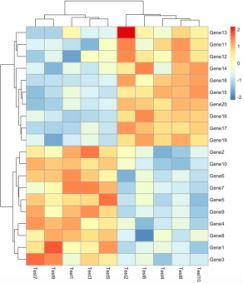```pheatmap(test, color = colorRampPalette(c("navy", "white", "firebrick3"))(50)) ```

# colorRampPalette函数可以设置3种颜色(只能是3种)，它可以根据给定的向量生成渐变色，这三个参数分别指定了最大值，中间值和最小值的颜色。绘图结果如下：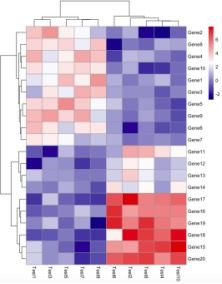```pheatmap(test, cluster_col = FALSE) ```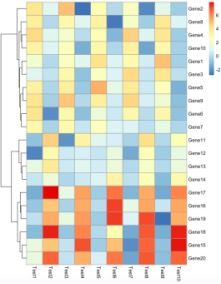show_rownames和show_colnames参数来控制是否显示行名和列名，如下：

```pheatmap(test,show_rownames=F,show_colnames=F) ```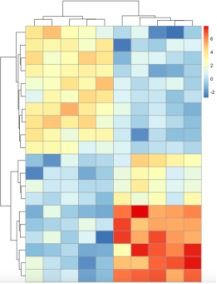display_numbers 和number_color 参数可以控制是否在图中显示数字及设置数字的颜色。

`pheatmap(test, display_numbers = TRUE,number_color = "blue")`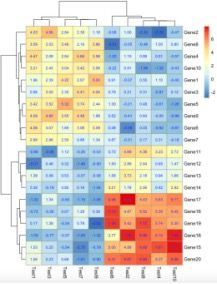cellwidth和cellheight两个参数可以控制每个单元的长度和宽度。参数main可以在图片中添加标题。

`pheatmap(test, cellwidth = 15, cellheight = 12, main = "Example heatmap")`